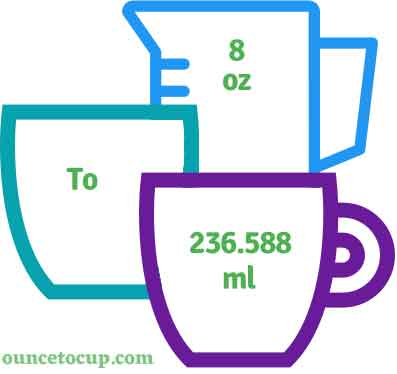# 8 oz to ml (8 ounce to Milliliter Conversion)

Are you cooking your favorite dish? The detailed chart in the recipe includes the calculation of 8 ounce to milliliter conversion? Do not worry; check this conversion tool to find how many 8 ounce equal to milliliter in a minute. This 8 ounce to milliliter converter gives an exact measurement for any recipe you prepare. No complexities here; just follow the steps to do a 8 ounce to milliliter conversion and get the precise answer for your cooking or baking recipes.

Input Here

oz

Output

ml

8 ounce = 236.588 Milliliter
(8 oz = 236.588 ml)

Try our auto 8 ounce to milliliter calculator(Without Convert Button), Just change the first field value 8 oz and you got final value ml.## How many ml in an 8 oz?

We know that the fractional value of 8 oz is equal to 236.588 ml. If you want to convert 8 fluid oz to an equal number of ml, just multiply the volume value by 29.5735. Hence, 8 ounce is equal to 236.588 ml.

The Answer is: 8 US Fluid Ounce = 236.588 US Milliliter

8 oz = 236.588 ml

Many of them try to search or find an answer for what is 8 ounces in ml? So, we’ll start with 8 fl oz to ml conversion to know how big is 8 oz.

## A simple formula to convert 8 fluid oz to ml:

We know that the fractional value of 8 oz is equal to 236.588 ml. If you want to convert fluid 8 oz to an equal number of milliliter, just follow the below steps to make your work easy.

Fluid 8 ounces to Milliliter formula

ml = 8 fl oz * 29.5735 where fl oz is Fluid Ounce

In the U.S Customary measurement system, the one unit 8 oz U.S volume was found to be equal to 236.588 U.S Milliliter. It is also represented as 8 US Fl Oz = 236.588 U.S Ml. It is also noted in this symbol as “fl oz”.

## Some quick chart for your fluid 8 ounce to Milliliter conversion are below:

 8 oz = 236.588 ml 9 oz = 266.162 ml 10 oz = 295.735 ml 11 oz = 325.309 ml 12 oz = 354.882 ml 13 oz = 384.456 ml 14 oz = 414.029 ml 15 oz = 443.603 ml 16 oz = 473.176 ml 17 oz = 502.75 ml

## How to convert 8 oz to ml?

• In the fractional value, 8 ounce (8 oz) is measured to 236.588 ml.
• It is also similar to the calculation of 8 Fluid ounce (8 oz) = 236.588 ml value If you want to convert 8 fluid oz to Ml
• Just multiply the fluid in 8 ounce i.e. 8 oz by 29.5735
• Applying to formula, ml = 8 ounce * 29.5735 = 236.588
• Hence, 8 ounce is equal to 236.588 ml.